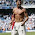# Class 10, NCERT (CBSE) Chemistry Notes and Sample (Hot) Questions | Chapter 1, Chemical Reactions and Equations

Ncert Cbse Class 10, Science (Chemistry)
Chemical Reactions and Equations
Science Notes
What is a ‘Reaction’?
Reaction is a term used for depicting a change or transformation in which a substance decomposes, combines with other substances, or interchanges constituents with other substances.
What is a ‘Chemical Reaction’?
A chemical change is always accompanied by a chemical reaction. a chemical change or reaction can be confirmed by any or all of the following observations:
=> change in state
=> change in colour
=> change in temperature
=> evolution of gas.
What is a ‘Chemical Equation’?
A Chemical Equation represents a chemical reaction. A complete chemical equation represents the reactants, products, and their physical states symbolically. A chemical equation also indicates a number of atoms of each element involved in a reaction. A chemical equation can be written as balanced or unbalanced. An unbalanced chemical equation is also known as Skeletal Chemical Equation for a reaction. An equation having an equal number of atoms of each element on both the sides is called a balanced chemical equation.
Types of Chemical Reaction
Chemical Reactions are primarily of five types as listed below:
1. Combination reaction
2. Decomposition reaction
3. Displacement reaction
4. Double displacement reactions
5. Oxidation and Reduction reactions
What are ‘Combination Reactions’?
When two or more substances (elements or compounds) combine to form a single product, the reactions are called ‘Combination Reactions’. Generally, Combination Reactions are exothermic in nature. The following are some examples of Combination Reactions:
CaO (s) + H2O (l) -----> Ca(OH)2 (aq)
C (s) + O2 (g) ------> CO2 (g)
What are ‘Decomposition Reactions’?
Decomposition reactions are opposite to combination reactions. In a Decomposition reaction, a single substance decomposes to give two or more substances.
What are ‘Displacement Reactions’?
When an element displaces another element from its compound, such reaction is called a displacement reaction e.g. -
Zn (s) + CuSO4 (aq) -----> ZnSO4 (aq) + Cu (s)
What are ‘Double Displacement Reactions’?
A double displacement reaction is a bimolecular process in which parts of two compounds are exchanged to give two new compounds. The general equation used to represent double displacement reactions can be written as -
AB + CD -----> AD + BC
Examples of double displacement reactions are -
Na2SO4 (aq) + BaCl2 (aq) -----> BaSO4 (s) + 2NaCl (aq)
“Double Displacement Reactions” have two commoj features -
1. Two compounds exchange their ions resulting in the formation of two new compounds.
2. One of the new compounds formed would be separated from the mixture in some way, commonly as a solid or gas.
Double displacement reactions can be further classified as Precipitation, Gas formation, and Acid-base neutralization reactions.
Sample Hot Questions
Q.1: Which of the following is a Combination and which is a Displacement Reaction?
(a) Cl2 + 2KI -----> 2KCl + I2
(b) 2K + Cl2 ------> 2KCl
Ans:
(a) Displacement reaction
(b) Combination reaction
Q.2: What is a catalytic decomposition?
Ans: Those reactions in which a compound splits up into two or more simpler substances in the presence of catalyst are called catalytic reactions. For example, digestion of food in human body.
Q.3: What is the term used for the symbolic representation of a reaction.
Ans: Chemical equation.
Q.4: Write two uses of decomposition reactions.
Ans: Two uses of decomposition reactions -
(i) These reactions are used to extract several metals from their oxides and salts. When the oxide or salt is electrolyzed, metal is obtained as per the following equation:
2Al2O3 ------> 4Al + 3O2
(ii) Digestion of food in our body is also an example of decomposition reaction. The starch (carbohydrates) present in the body is decomposed to give simple substance (glucose) and the proteins are broken down into amino acids.
Q.5: Explain the terms ‘oxidizing agent’ and reducing agent’.
Ans:
Oxidizing agent
The substance, which oxidizes elements or compounds, is known as oxidizing agent e.g. -
4Fe + 3O2 ------> 2Fe2O3
Hence, oxygen is an oxidizing agent. In this equation it oxidizes iron (FeII) to ferric oxide (Fe2O3).
Reducing agent
The substance, which oxidizes elements or compounds, is known as oxidizing agent e.g. -
SnCl2 + 2FeCl3 -----> SnCl4 + 2FeCl2
Here stannous chloride is a reducing agent. It reduces ferric chloride to ferrous chloride.
Further Study on Chapter 1, Chemical Reactions and Equations

##### 1 comment:
1.